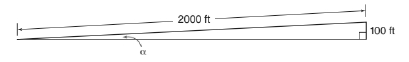Chapter 11.1, Problem 30EElementary Geometry For College St...

7th Edition
Alexander + 2 others
ISBN: 9781337614085

Solutions

Chapter
SectionElementary Geometry For College St...

7th Edition
Alexander + 2 others
ISBN: 9781337614085
Textbook Problem

In Exercises 27 to 34, use the drawings, where provided, to solve each problem. Angle measures should be given to the nearest degree; distances should be given to the nearest tenth of a unit.Over a 2000-ft span of highway through a hillside, there is a 100-ft rise in the roadway. What is the measure of the angle formed by the road and the horizontal?To determine

To find:

The angle formed by the road and the horizontal.

Explanation

In a right angle triangle,

1) The side PQ, which is opposite to the right angle PRQ is called the hypotenuse.

(The hypotenuse is the longest side of the right triangle.)

2) The side RQ is called the adjacent side of angle θ.

3) The side PR is called the opposite side of angle θ.

Hence, the sine ratio for an acute angle is oppositehypotenuse

Given:

From, the given diagram,

Opposite side = 100 ft and hypotenuse = 2000 ft

Let α be the angle formed by the road and the horizontal

Still sussing out bartleby?

Check out a sample textbook solution.

See a sample solution

The Solution to Your Study Problems

Bartleby provides explanations to thousands of textbook problems written by our experts, many with advanced degrees!

Get Started

In Exercises 73-80, find the indicated limits, if they exist. 75. limx3x3+x2+1x3+1

Applied Calculus for the Managerial, Life, and Social Sciences: A Brief Approach

Subtract: 4000484

Elementary Technical Mathematics

For f(x)=7+5x2x2, find and simplify f(x+h)f(x)h

Mathematical Applications for the Management, Life, and Social Sciences

The fourth term of {an} defined by a1 = 3, and for n = 1, 2, 3, … is:

Study Guide for Stewart's Single Variable Calculus: Early Transcendentals, 8th